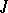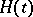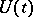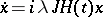# Multipliers

(diff) ← Older revision | Latest revision (diff) | Newer revision → (diff)

of the first and second kinds

The eigen values of the monodromy operator of a canonical equation.

In a complex Hilbert space, equations of the form, whereandare self-adjoint operators,andis periodic, are called canonical. In the finite-dimensional case the eigen values of the monodromy operatorof this equation are called multipliers. If all solutions of a canonical equation are bounded on the entire real axis (the equation is stable), then the multipliers lie on the unit circle. Consider a canonical equationwith a real parameter; then all multipliers can be divided into two groups: multipliers of the first (second) kind, which move counter-clockwise (clockwise) asincreases.

A canonical equation is called strongly stable if it is stable and remains stable under small variations of. For strong stability it is necessary and sufficient that all multipliers be on the unit circle and that there be no coincident multipliers of different kinds.

The theory of multipliers of the first and second kinds allows one to obtain a number of delicate tests for stability and estimates of the zone of stability for canonical equations. The homotopy classification of stable and unstable canonical equations has been given in terms of multipliers.

How to Cite This Entry:
Multipliers. Encyclopedia of Mathematics. URL: http://encyclopediaofmath.org/index.php?title=Multipliers&oldid=13903
This article was adapted from an original article by S.G. Krein (originator), which appeared in Encyclopedia of Mathematics - ISBN 1402006098. See original article